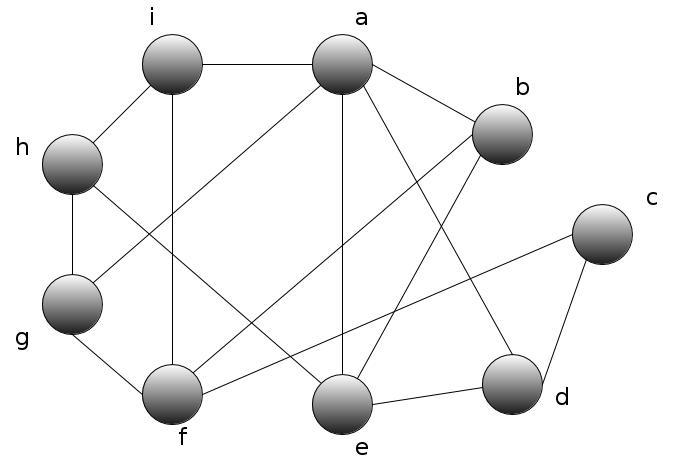# Planar graph check

kljoki
Hi
I have this graphnow i should check if this graph can be planar.

v - number of vertices
e - number of edges
f - number of faces

to be planar it should hold v - e + f = 2 from here f = 2 - v + e = 2 - 9 + 15 = 8
so f = 8 now my question is how to easily count faces (regions bounded by edges, including the outer, infinitely large region) of the graph??
thanks

Mentor
couldnt you start with point a and then compile a list of connections that come back to a

a-b-e

a-b-f-i

and continue to point b, c, d ... making sure not to count the same loop again

as an example b-e-a is the same as a-b-e

Hi
I have this graph
View attachment 50059

now i should check if this graph can be planar.

v - number of vertices
e - number of edges
f - number of faces

to be planar it should hold v - e + f = 2 from here f = 2 - v + e = 2 - 9 + 15 = 8
so f = 8 now my question is how to easily count faces (regions bounded by edges, including the outer, infinitely large region) of the graph??
thanks

How about arguing that your graph contains neither a K3,3 nor a K5

as a subgraph? it seems there are not that many cases to consider...